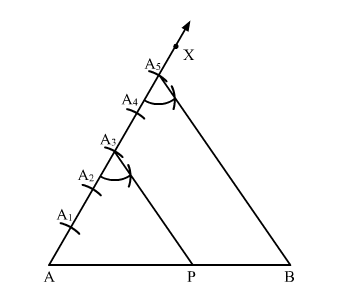# Draw a line segment AB of length 7 cm.`
Question:

Draw a line segment $A B$ of length $7 \mathrm{~cm}$. Using ruler and compasses, find a point $P$ on $A B$ such that $\frac{A P}{A B}=\frac{3}{5}$.

Solution:

Steps of Construction:

Step 1. Draw a line segment AB = 7 cm.

Step 2. Draw a ray AX, making an acute angle ">BAX.

Step 3. Along AX, mark 5 points (greater of 3 and 5)  A1, A2, A3, A4 and A5 such that

AA= A1A2 = A2A= A3A4 = A4A5

Step 4. Join A5B.

Step 5. From A3, draw A3P parallel to A5B (draw an angle equal to ">AA5B), meeting AB in P.Here, $\mathrm{P}$ is the point on $\mathrm{AB}$ such that $\frac{\mathrm{AP}}{\mathrm{PB}}=\frac{3}{2}$ or $\frac{\mathrm{AP}}{\mathrm{AB}}=\frac{3}{5}$.